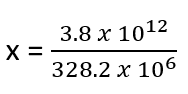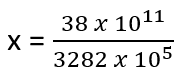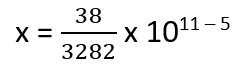# Math Worksheets Land

Math Worksheets For All Ages

# Math Worksheets Land

Math Worksheets For All Ages

# Scientific Notation Word Problems Worksheets

What is Scientific Notation? How wonderful it is to find out that you can write short forms instead of going on writing long digit numbers. You should be thankful for the discovery of scientific notation concepts; you can save the effort of writing continuously long digits. To put it simply, scientific notation is writing the short forms of very long numbers. Instead of writing the number in decimal form, scientific notation helps in making the short. The number is multiplied by the power of ten and shortened. In mathematical notation, the first number is the 'coefficient.' It is always greater than or equal to one and less than 10. For instance, to write the scientific notation for 256, the coefficient has to be shortened to 2.56 However, the second number in the equation is expressed in the form of a power of 10, written as 10 with an exponent. Combining these two numbers, you will get a kind of equation like this. 2.56 x 102. In case you see a negative exponent, it shows that the decimal has to be moved to the left.

### Aligned Standard: Grade 8 Expressions and Equations - 8.EE.A.4

• Population Problem Step-by-Step Lesson- We do a little scientific notation subtraction to solve this one. Very basic at first.
• Guided Lesson - The mass of the Earth and the Sun get in the mix; along with another population problem.
• Independent Practice - These are such random questions. I did Google to find actual realistic numbers for them. Who really figured out how much a dust particle weighed?
• Matching Worksheet -Match the circumstances to their outcomes. You will find these problems a bit challenging to step up.
• Answer Keys - These are for all the unlocked materials above.

### Homework Sheets

When students first see the length of problems like this they are intimidated.

• Homework 1 - In 2010, there were approximately 6 million girls living in Orange City. In 2011, the population was down to 4 million girls. How many girls lived in Orange City in 2011 in comparison to 2010? Write your answer in scientific notation.
• Homework 2 - Get them into the same power of ten to make it workable. A nice way to do that is to move the decimal one to the right on Lake City’s population. This results in losing a power of ten.
• Homework 3 - Suppose there are 3 × 106 fish in every 2 km area of sea. How many fish are there in 6 km of sea?

### Practice Worksheets

Once students master the art of outlining, these problems become much easier for them.

• Practice 1 - 0.000078 is the wave length of white light. Can you express the measurement using scientific notation?
• Practice 2 - In India, the population was 500 million people in 2000. The total health care costs for the country at that time were \$ 2.5 trillion. Calculate the average amount spent per person on health care.
• Practice 3 - wo men are working together on a project. The first man earns 3 x 105dollars and the second one earns 3 x 104 dollars for the project. How much more money does the first man earn?

### Math Skill Quizzes

Remind students that when the tail ends of scientific notation is the same, they cancel.

• Quiz 1 - A robbery in London resulted in 6,040,000,000 pounds being stolen. Write this number in scientific notation.
• Quiz 2 - If the population of Vatican City spends 40 million Euros in 40 days, find the amount spent in 15 days.
• Quiz 3 - Microwave ovens work at a wave frequency of 3 x 103 MHz. What will be the wave frequency if we use 1/3 of the frequency?

### Example: Scientific Notation Word Problem

Problem: In 2019, the U.S. population was calculated to be 328.2 million people by the national Census Bureau. In that same year the healthcare cost of that nation were \$3.8 trillion dollars. Determine the cost of healthcare per person in the nation at that time?

Solution: To determine this value we will need to divide the total cost (\$3.8 trillion) by the number of people (328.2 million). But first we need to get these values into a workable format. This is where our powers of ten and scientific notation come in handy.

Step 1) Convert the Values to Scientific Notation

A million can be expressed as 106. So, 328.2 million can be expressed as: 328.2 x 106.

A trillion can be expressed as 1012. So, 3.8 trillion can be expressed as: 3.8 x 1012.

Step 2) Divide Cost by the Number of People

We just have to setup the math. We would place our values that are now in scientific notation into the original quotient that we were trying to determine. We can call this unknown cost per person the variable x. So, we would write it as:We can remove those decimal values by dropping a power of ten for each decimal we remove. In this case there is only one decimal value in each. So, this can be rewritten as:We can then rewrite this as a fraction multiplied by a combined power of ten, as you will see below:Convert the fraction to a decimal and combine the powers of ten:

x = 0.0116 x 106

We state this value as dollar figure to answer our problem:

0.0116 x 106 = \$11,600.

This means that the United States spent \$11,600 per person on healthcare in 2019.

Unlock all the answers, worksheets, homework, tests and more!
Save Tons of Time! Make My Life Easier Now

## Thanks and Don't Forget To Tell Your Friends!

I would appreciate everyone letting me know if you find any errors. I'm getting a little older these days and my eyes are going. Please contact me, to let me know. I'll fix it ASAP.